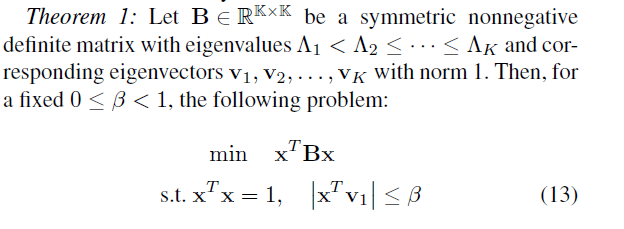# Absolute function is convex? and 2^x can use cvx?1. i have wonder ,if x is a variable vector ,v1is constant vector,absolute | x^T*v1| is cnvex?
2. x is a variable vector,2^x can use cvx?
1. help cvx/abs
`````` Disciplined convex/geometric programming information for abs:
abs(X) is convex and nonmonotonic in X. Therefore, according to
the strict rules of DCP, X must be affine. However, because of
its special structure, CVX considers the sign of X as well. So,
for instance, if X is known to be nonnegative, then abs(X)=X.
``````

`x' * v1` is affine (as a function of x) if v1 is constant. Therefore, abs(x’ * v1) is convex and is formed in accordance with CVX’s rules, and therefore
`abs(x' * v1) <= beta`
will be accepted by CVX.

1. `2^x` can be re-written as `exp(x*log(2))` , which will be accepted by CVX. CVX is smart enough to apparently do this transformation and accept `2^x directly`, although I don’t see the rule in the CVX Users’ Guide which says that it will do so. I will let @mcg either acknowledge the opportunity to add such mention to the Users’ Guide or point out which rule therein covers this.

Thank you very much for your help, i have wonder,for 2^x ,could i re-written as power(2,x) in cvx ?

Yes, you are right. There are two forms of power. The first form has the first argument be a CVX expression and the second argument a constant. The second form has the first argument be a constant and the second argument a CVX expression. This second form is documented as follows in the CVX Users’ Guide.

power(p,x) p.^x and p^x, where p is a real constant and x is a real variable. For p^x, both p and x
must be scalars. Valid values of p include:
• p element of {0,1} Constant.
• 0 < p < 1. Convex and nonincreasing; x must be concave.
• p > 1. Convex and nondecreasing; x must be convex.
Negative values of p are not permitted.

That CVX will directly accept p^x, rather than requiring use of power(p,x), appears to be undocumented (or at least I can’t find it).

you are so kind ,thank you

Well, the formatting may be improvable but you quoted the very section that says you can use `p^x` and `p.^x` directly…